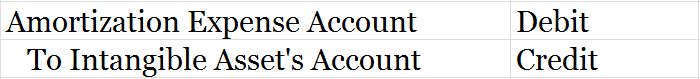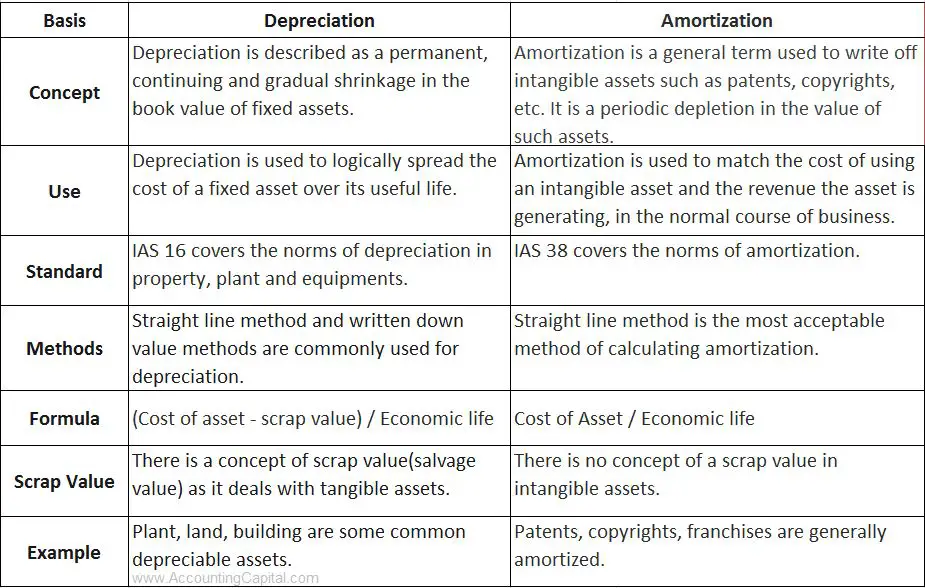# What is the Difference Between Depreciation and Amortization?

## Depreciation vs Amortization

One of the main principles of accrual accounting is that an asset’s cost is proportionally expensed based on the period over which it is used. Both depreciation and amortization (as well as depletion and obsolescence) are methods that are used to reduce the cost of a specific type of asset over its useful life. This article describes the main difference between depreciation and amortization.

Depreciation is for tangible fixed assets whereas amortization is for intangible assets, however, in a way they are similar yet different at the same time. We have compared depreciation and amortization in 5 distinct points below;

## Depreciation

1. Reduction in the value of a tangible asset due to normal usage, wear and tear, new technology, or unfavourable market conditions is called depreciation. Assets such as plant and machinery, buildings, vehicles, etc. which are expected to last more than one year, but not for an infinite number of years are subject to depreciation.

2. It only applies to fixed tangible assets.

3. Straight line, Diminishing value, etc. are a few of the various methods to charge depreciation.

4. Journal entry for depreciation

5. Example of Depreciation

Machinery cost  = 10,000, Depreciation Rate = 20%

= 20/100*10,000

Depreciation charge (1st year) = 2,000

## Amortization

1. The process of spreading the cost of an intangible asset such as patent, copyright, trademark, etc. over a specific period i.e. equal to the course of its useful life is called Amortization.

2. It only applies to intangible assets.

3. Only the Straight-line method is used for the amortization of intangible assets.

4. Journal entry for amortization*without using accumulated amortization account

5. Example of Amortization

Patent cost = 10,000, Useful life of patent = 10 years

Amortization rate/year = 10%

1st year = 10/100*10,000

Amortization expense (1st year) = 1,000

Demonstrated above are the major points of difference between depreciation and amortization along with their respective examples.

Related Topic – Asset Disposal Account

## Difference Between Depreciation & Amortization (Table Format)Related Topic – Difference between Tangible and Intangible Assets

## Types of Depreciation and Amortization

Types of Depreciation

These types of depreciation are mandated by law and enforced by professional accounting practices all over the world.

• Straight-line method: Under this method, depreciation is calculated on the original cost of the asset after deducting the scrap value. Depreciation is charged every year and at the end of the life of the asset, the value of the asset becomes zero. The amount of depreciation is the same every year.
• Written down value method: The rate of depreciation is fixed in this method, but depreciation at this rate is calculated on the balance of the asset standing in the books on the first day of each accounting year. It is suitable for assets with high repair charges. It is also known as
• Annuity method: Under this method, the amount of depreciation includes some portion of the expected amount of interest also. This interest is the forgone expected interest that the purchaser would have earned had he invested the cost of the asset somewhere else.
• Depreciation Fund Method: Under this method, an amount equal to the annual depreciation is charged against the profits every year and accumulated in the form of a sinking fund. This amount is invested to earn interest and used to replace the asset after the end of its useful life.
• Group Depreciation: Under this method, the value of homogeneous assets is totalled up and from this total, the residual value of all the assets is deducted and the balance is found out. This balance is divided by the average of the estimated lives of all these assets and the result is the depreciation for one year.

Types of Amortization

Types of amortization usually refer to the various methods of amortization of a loan schedule. This finds more use in finance management than general accounting.

• Straight-line amortization: A constant amortization method where the principal remains constant, but the interest changes with the outstanding obligation. The instalment amount keeps changing.
• Mortgage style amortization: Also known as a constant payment method. The instalment amount does not change, but the principal and the interest keep changing.
• Interest-only amortization: A different kind of setup where the principal is paid in a lump sum after the amortization schedule expires.
• Line of credit amortization: It works like a revolving letter of credit. The amount is withdrawn in the draw period and repaid in the repayment period.

Related Topic – Adjustments in Final Accounts

## Is goodwill depreciated or amortized?

Goodwill is an intangible fixed asset. It is created through a process that carries a certain value but can not be seen or touched. It is an attractive force that results in additional profits and/or value creation. Its value depends on factors like popularity, image, prestige, honesty, fairness, etc.

Nonetheless, it is an asset and hence its cost has to match up with the revenue it generated in a particular accounting year. Since goodwill is an intangible asset, its value has to be amortized. But, in a disruptive decision of 2001, the Financial Accounting Standards Board (FASB) disallowed the amortization of goodwill as an intangible asset.

The reason was that most companies use the purchase method to evaluate and record the amount of goodwill in their books since goodwill comes into the picture when a new business is purchased, or a new asset is purchased. This happens when a company pays more than the fair value of an asset.

FASB allowed the evaluation for impairment, annually for goodwill. Impairment evaluation is a complex and costly process, so the FASB reallowed the amortization of goodwill as an intangible asset over 10 years in 2014, only for private companies.

Goodwill can be amortized in the following way:

ABC Ltd is purchasing a smaller company X that has a net worth of 450 million. But, X enjoys a reputation in the niche local market so the purchase consideration was fixed at 500 million. After doing a thorough revaluation, the accountants found the fair value of X assets to be 470 million.

ABC Ltd records goodwill as (purchase consideration – net worth) = 50 million

The amortization amount is (book value of assets – fair value of assets) = 30 million

Now, goodwill in the books of ABC Ltd will be recorded at (50 million – 30 million) = 20 million

While the amortized goodwill of 30 million will be spread over 10 years at 3 million per year. This amount will be charged to the profit & loss account for 10 years.